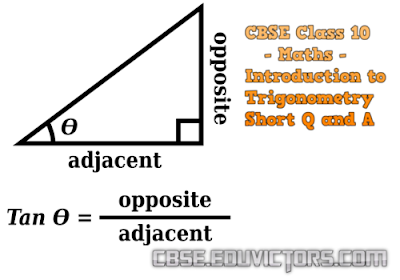## Pages

Showing posts with label Trigonometry. Show all posts
Showing posts with label Trigonometry. Show all posts

## Introduction to Trigonometry

Short Q and A

Q1: If sin 3A = cos (A – 26°), where 3A is an acute angle, find the value of A.

Answer: sin 3A = cos (A – 26°)

∵ sin 3A = cos (90° – 3A)
∴ cos (90° – 3A) = cos (A – 26°)

Since
90° – 3A and A – 26° are both acute angles, therefore,
90° – 3A = A – 26°
A = 29°

Q2: Express cot 85° + cos 75° in terms of trigonometric ratios of angles between 0° and 45°.

Answer: cot 85° + cos 75° = cot (90° – 5°) + cos (90° – 15°)

= tan 5° + sin 15°